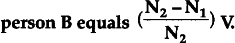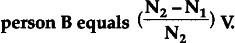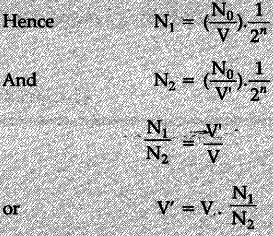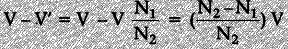# A given number of atoms, N0 of a radioactive element with a half life

A given number of atoms, N0 of a radioactive element with a half life T is uniformly distributed in the blood stream of a
(i) normal person A having total volume V .of blood in the body
(ii) person B in need of blood transfusion having a volume V’ of blood in the body.
The number of radioactive atoms per unit volume in the blood streams of the two persons after a time { n }_{ 1 }
are found to be
{ N }_{ 2 }
and
{ N }_{ 2 }.
Prove mathematically that the additional volume of blood that needs to be transfused in the body ofInitial number of radioactive atoms, per unit volume, in the blood streams of persons A and B areAfter a time nT (T = Half-life), these numbers would get reduced by a factor 2".Additional volume of blood needed by person B is